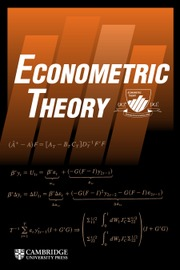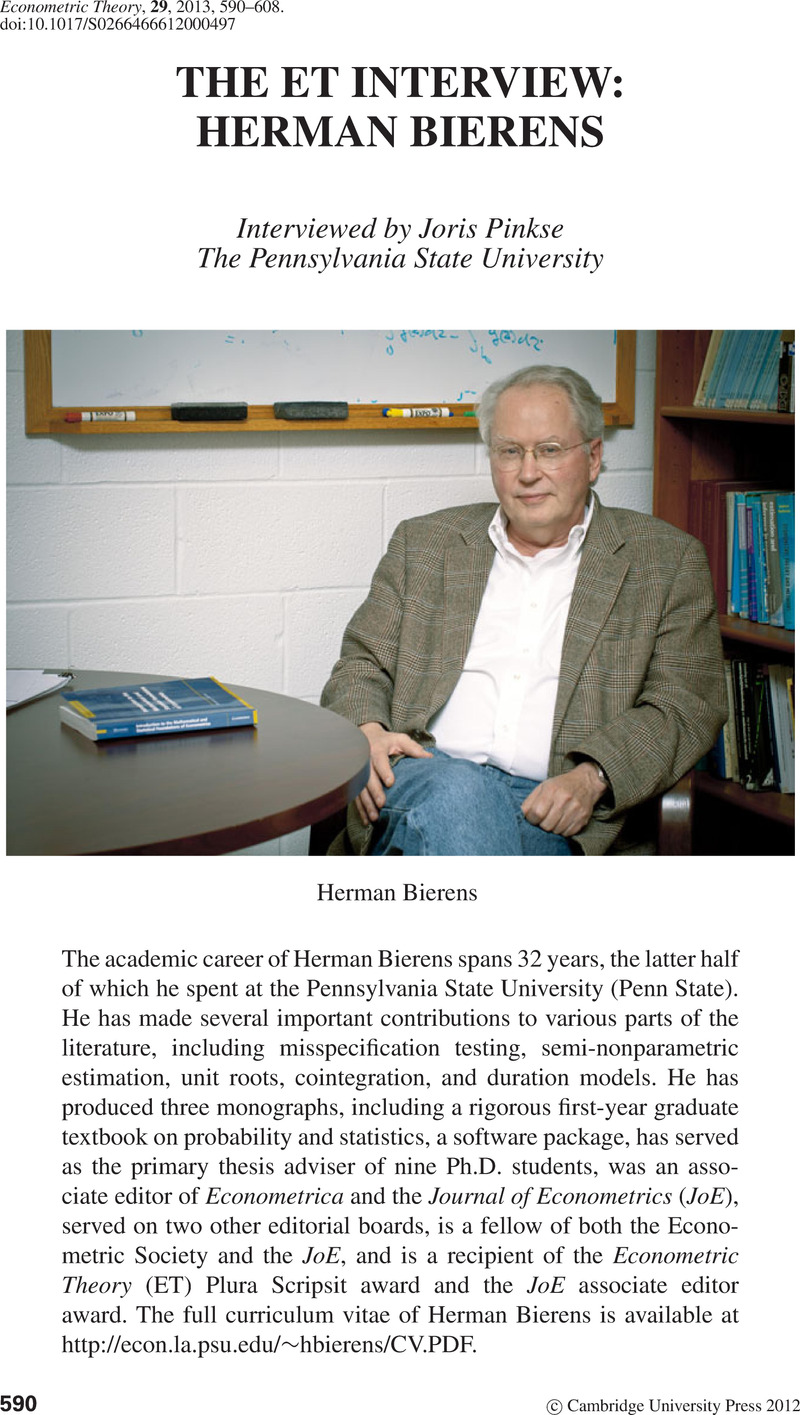Home
Hostname: page-component-7f7b94f6bd-gszfc Total loading time: 0.481 Render date: 2022-06-30T06:53:30.038Z Has data issue: true Feature Flags: { "shouldUseShareProductTool": true, "shouldUseHypothesis": true, "isUnsiloEnabled": true, "useRatesEcommerce": false, "useNewApi": true } hasContentIssue trueEconometric Theory

## AbstractType
ET Interview
Information
Econometric Theory , June 2013 , pp. 590 - 608

## Access options

Get access to the full version of this content by using one of the access options below. (Log in options will check for institutional or personal access. Content may require purchase if you do not have access.)

## References

Andrews, D. (1997) A conditional Kolmogorov test. Econometrica 65, 10971128.CrossRefGoogle Scholar
Bierens, H. (1980) Robust methods and asymptotic theory in nonlinear econometrics. Ph.D. thesis, University of Amsterdam.CrossRefGoogle Scholar
Bierens, H. (1981) Robust Methods and Asymptotic Theory in Nonlinear Econometrics. Springer-Verlag.CrossRefGoogle Scholar
Bierens, H. (1982) Consistent model specification tests. Journal of Econometrics 20, 105134.CrossRefGoogle Scholar
Bierens, H. (1983) Uniform consistency of kernel estimators of a regression function under generalized conditions. Journal of the American Statistical Association 78, 699707.CrossRefGoogle Scholar
Bierens, H. (1984) Model specification testing of time series regression. Journal of Econometrics 26, 323353.CrossRefGoogle Scholar
Bierens, H. (1987) Kernel estimators of regression functions. In Truman Bewley, (ed.), Advances in Econometrics: Fifth World Congress, vol. I, pp. 99144.CrossRefGoogle Scholar
Bierens, H. (1990) A consistent conditional moment test of functional form. Econometrica 58, 14431458.CrossRefGoogle Scholar
Bierens, H. (1993) Higher–order sample autocorrelations and the unit root hypothesis. Journal of Econometrics 57, 137160.CrossRefGoogle Scholar
Bierens, H. (1994) Topics in Advanced Econometrics: Estimation, Testing, and Specification of Cross-Section and Time Series Models. Cambridge University Press.CrossRefGoogle Scholar
Bierens, H. (1997) Nonparametric cointegration analysis. Journal of Econometrics 77, 379404.CrossRefGoogle Scholar
Bierens, H. (1997) Testing the unit root with drift hypothesis against nonlinear trend stationarity, with an application to the U.S. price level and interest rate. Journal of Econometrics 81, 2964.CrossRefGoogle Scholar
Bierens, H. (2001) Complex unit roots and business cycles: Are they real? Econometric Theory 17, 962983.CrossRefGoogle Scholar
Bierens, H. (2004) Introduction to the Mathematical and Statistical Foundations of Econometrics. Cambridge University Press.CrossRefGoogle Scholar
Bierens, H. (2007) Econometric analysis of linearized singular dynamic stochastic general equilibrium models. Journal of Econometrics 236, 595627.CrossRefGoogle Scholar
Bierens, H. (2008) Semi-nonparametric interval-censored mixed proportional hazard models: Identification and consistency results. Econometric Theory 24, 749794.CrossRefGoogle Scholar
Bierens, H. (2012) Consistency and Asymptotic Normality of Sieve estimators under Weak and Verifiable Conditions. Technical report, Penn State.Google Scholar
Bierens, H. & Broersma, L. (1993) The relation between unemployment and interest rate: Some empirical evidence. Econometric Reviews 12, 217256.CrossRefGoogle Scholar
Bierens, H. & Carvalho, J. (2007) Semi-nonparametric competing risks analysis of recidivism. Journal of Applied Econometrics 22, 971993.CrossRefGoogle Scholar
Bierens, H. & Carvalho, J. (2011) Job search, conditional treatment and recidivism: The employment services for ex-offenders program reconsidered. B.E. Journal of Economic Analysis & Policy 11(1–5).CrossRefGoogle Scholar
Bierens, H. & Hartog, J. (1988) Estimating a hedonic earnings function with a nonparametric method. Empirical Economics 13, 267294.Google Scholar
Bierens, H. & Hartog, J. (1988) Non-linear regression with discrete explanatory variables, with an application to the earnings function. Journal of Econometrics 38, 269299.CrossRefGoogle Scholar
Bierens, H. & Martins, L. (2010) Time varying cointegration. Econometric Theory 26, 14531490.CrossRefGoogle Scholar
Bierens, H. & Ploberger, W. (1997) Asymptotic theory of integrated conditional moment tests. Econometrica 65, 11291151.CrossRefGoogle Scholar
Bierens, H. & Song, H. (2012) Semi-nonparametric estimation of independently and identically repeated first-price auctions via an integrated simulated moments method. Journal of Econometrics 168, 108119.CrossRefGoogle Scholar
Bierens, H. & Swanson, N. (2000) The econometric consequences of the ceteris paribus condition in economic theory. Journal of Econometrics 95, 225253.CrossRefGoogle Scholar
Bierens, H. & Wang, L. (2012) Integrated conditional moment tests for parametric conditional distributions. Econometric Theory 28, 328362.CrossRefGoogle Scholar
Billingsley, P. (1968) Convergence of Probability Measures. Wiley.Google Scholar
Billingsley, P. (2012) Probability and Measure. Wiley.Google Scholar
Breitung, J. (2002) Nonparametric tests for unit roots and cointegration. Journal of Econometrics 108, 343363.CrossRefGoogle Scholar
Escanciano, J.C. (2006) A consistent diagnostic test for regression models using projections. Econometric Theory 22, 10301051.CrossRefGoogle Scholar
Gallant, A.R. (1997) An Introduction to Econometric Theory: Measure-Theoretic Probability and Statistics with Applications to Economics. Princeton University Press.CrossRefGoogle Scholar
Gallant, A.R. & Nychka, D. (1987) Semi-nonparametric maximum likelihood estimation. Econometrica 55, 363390.CrossRefGoogle Scholar
Gallant, A.R. & Tauchen, G. (1989) Seminonparametric estimation of conditionally constrained heterogeneous processes: Asset pricing applications. Econometrica 57, 10911120.CrossRefGoogle Scholar
Guerre, E., Perrigne, I., & Vuong, Q. (2000) Optimal nonparametric estimation of first price auctions. Econometrica 68, 525574.CrossRefGoogle Scholar
Heckman, J. & Honoré, B. (1989) The identifiability of the competing risks model. Biometrika 76, 325330.CrossRefGoogle Scholar
Phillips, P. (1987) Time series regression with a unit root. Econometrica 55, 277301.CrossRefGoogle Scholar
Phillips, P. & Durlauf, S. (1988) Trends versus random walks in time series analysis. Econometrica 56, 13331354.Google Scholar
Phillips, P. & Perron, P. (1988) Testing for a unit root in time series regression. Biometrika 75, 335346.CrossRefGoogle Scholar
Powell, J.L., Stock, J., & Stoker, T. (1989) Semiparametric estimation of index coefficients. Econometrica 57, 14031430.CrossRefGoogle Scholar
Stinchcombe, M. & White, H. (1998) Consistent specification testing with nuisance parameters present only under the alternative. Econometric Theory 14, 295325.CrossRefGoogle Scholar
Stokey, N., Lucas, R., & Prescott, E. (1989) Recursive Methods in Economic Dynamics. Harvard University Press.CrossRefGoogle Scholar
Stute, W. (1997) Nonparametric model checks for regression. Annals of Statistics 25, 613641.Google Scholar
Zheng, J. (2000) A consistent test of conditional parametric distributions. Econometric Theory 16, 667691.CrossRefGoogle Scholar

# Save article to Kindle

Note you can select to save to either the @free.kindle.com or @kindle.com variations. ‘@free.kindle.com’ emails are free but can only be saved to your device when it is connected to wi-fi. ‘@kindle.com’ emails can be delivered even when you are not connected to wi-fi, but note that service fees apply.

Find out more about the Kindle Personal Document Service.

THE ET INTERVIEW: HERMAN BIERENS
Available formats
×

# Save article to Dropbox

To save this article to your Dropbox account, please select one or more formats and confirm that you agree to abide by our usage policies. If this is the first time you used this feature, you will be asked to authorise Cambridge Core to connect with your Dropbox account. Find out more about saving content to Dropbox.

THE ET INTERVIEW: HERMAN BIERENS
Available formats
×

# Save article to Google Drive

To save this article to your Google Drive account, please select one or more formats and confirm that you agree to abide by our usage policies. If this is the first time you used this feature, you will be asked to authorise Cambridge Core to connect with your Google Drive account. Find out more about saving content to Google Drive.

THE ET INTERVIEW: HERMAN BIERENS
Available formats
×
×